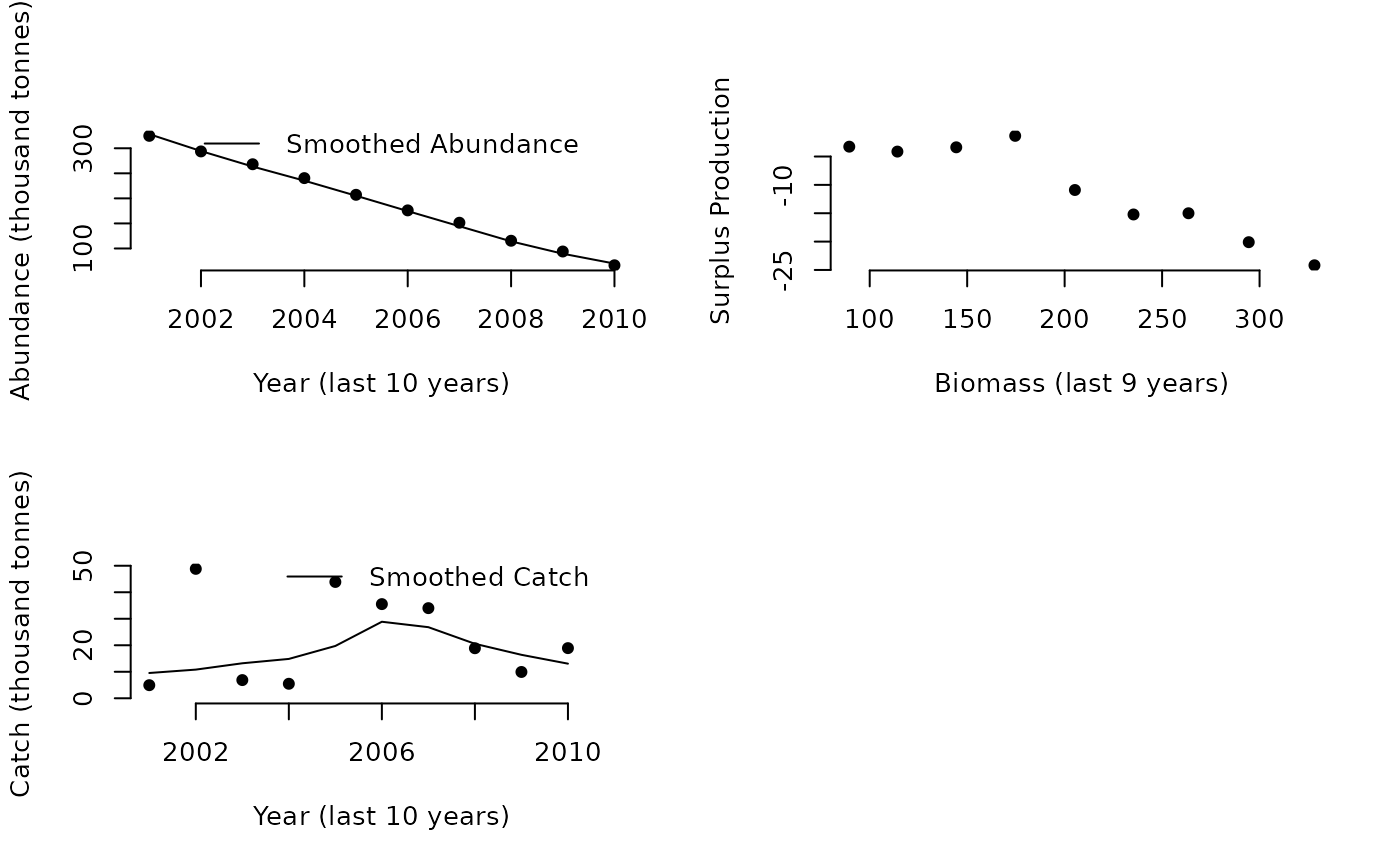A harvest control rule proposed by Carl Walters that uses trajectory in inferred surplus production to make upward/downward adjustments to TAC recommendations

Gcontrol(
x,
Data,
reps = 100,
plot = FALSE,
yrsmth = 10,
gg = 2,
glim = c(0.5, 2)
)

## Arguments

x

A position in the data object

Data

A data object

reps

The number of stochastic samples of the MP recommendation(s)

plot

Logical. Show the plot?

yrsmth

The number of years over which to smooth catch and biomass data

gg

A gain parameter

glim

A constraint limiting the maximum level of change in quota recommendations

## Value

An object of class Rec-class with the TAC slot populated with a numeric vector of length reps

## Details

The TAC is calculated as: $$\textrm{TAC} = \textrm{SP} \left(1-gG\right)$$ where $$\textrm{SP}$$ is the predicted surplus production for the next year, g is a gain parameter, and G is the slope of surplus production as a function of biomass over the last yrsmth years.

The change in TAC is bounded by the glim argument, which by default does not allow the TAC to decrease by more than half or increase more than twice the last annual catch.

## Required Data

See Data-class for information on the Data object

Gcontrol: Abun, Cat, Ind, Year

## Rendered Equations

See Online Documentation for correctly rendered equations

Other Index methods: GB_slope(), GB_target(), ICI(), Iratio(), Islope1(), Itarget1_MPA(), Itarget1(), ItargetE1()

## Author

C. Walters and T. Carruthers

## Examples

Gcontrol(1, Data=MSEtool::Atlantic_mackerel, plot=TRUE)#> TAC (median)
#>     6.529254